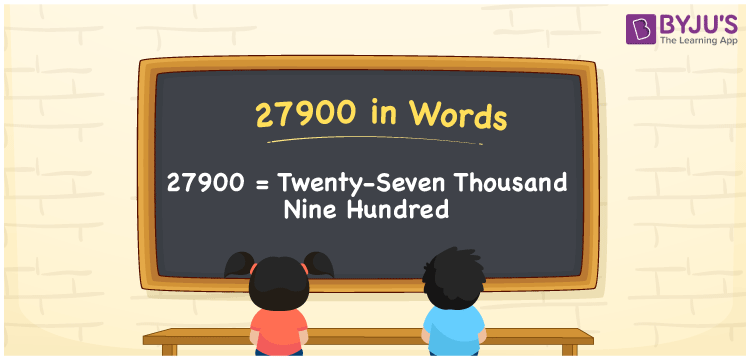# 27900 in Words

27900 in words is written as “Twenty-Seven Thousand Nine Hundred”. For example, Meera wrote a cheque of Rupees “Twenty-Seven Thousand Nine Hundred only” to help her friend. In Mathematics, 27900 is a cardinal number, that represents the quantity or value of something. 27900 can be also expressed as the sum of 27000 and 900, i.e.,27000 + 900 = 27900. Let us see in this article how to write 27900 in words using the place value chart.

 27900 in Words Twenty-Seven Thousand Nine Hundred Twenty-Seven Thousand Nine Hundred in Numbers 27900

## 27900 in English Words## How to Write 27900 in Words?

27900 is a five-digit number that can be written in words, by knowing the place value of each digit in the number. This can be done using a place value chart.

 Ten  Thousands Thousands Hundreds Tens Ones 2 7 9 0 0

We can see, from the above table, the positions of digits are:

• 2 → Ten Thousands
• 7 → Thousands
• 9 → Hundreds
• 0 → Tens
• 0 → Ones

Hence, when we read the number from right to left, it is Twenty-Seven Thousand Nine Hundred. Learn more about numbers in words at BYJU’S.

### Expanded Form of 27900

We can write the expanded form as:

2 × Ten thousand + 7 × Thousand + 9 × Hundred + 0 × Ten + 0 × One

= 2 × 10000 + 7 × 1000 + 9 × 100 + 0 × 10 + 0 × 1

= 20000 + 7000 + 900 + 0 + 0

= 27900

= Twenty-Seven Thousand Nine Hundred

27900 is the natural number that is succeeded by 27899 and preceded by 27901. Learn more about the number 27900 below:

• 27900 in Words – Twenty-Seven Thousand Nine Hundred
• Is 27900 an odd number? – No
• Is 27900 an even number? – Yes
• Is 27900 a perfect square number? – No
• Is 27900 a perfect cube number? – No
• Is 27900 a prime number? – No
• Is 27900 a composite number? – Yes

## Frequently Asked Questions on 27900 in words

Q1

### What is 27900 in words?

27900 in words is expressed as Twenty-Seven Thousand Nine Hundred.
Q2

### What is the place value of 7 in 27900?

The place value of 7 in 27900 is Thousands.
Q3

### How to spell 27900 in English words?

27900 in English is spelt as Twenty-Seven Thousand Nine Hundred